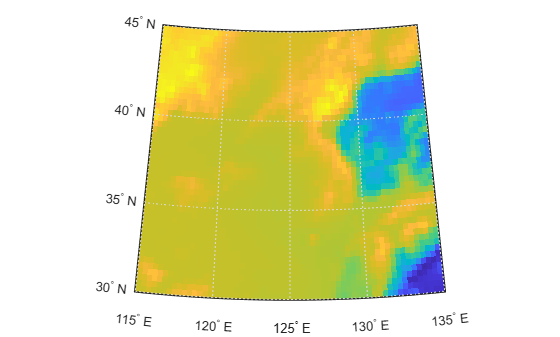# georesize

Resize geographic raster

## Syntax

``[B,RB] = georesize(A,RA,scale)``
``[B,RB] = georesize(A,RA,latscale,lonscale)``
``[B,RB] = georesize(___,method)``
``[B,RB] = georesize(___,'Antialiasing',TF)``

## Description

example

````[B,RB] = georesize(A,RA,scale)` returns the raster `B` that is `scale` times the size of the raster `A`. `RA` is a geographic raster reference object that specifies the location and extent of data in `A`. `georesize` returns the raster reference object `RB` that is associated with the returned raster `B`. By default, `georesize` uses cubic interpolation.`georesize` preserves the limits of the raster. If the scale specified divides evenly into the numbers of cells in each dimension, or the number of samples in each dimension minus 1, the limits of the output are the same as the input. Otherwise, `georesize` adjusts the output limits by a fraction of the cell extents or sample spacing values.```
````[B,RB] = georesize(A,RA,latscale,lonscale)` returns the raster `B` that is `latscale` times the size of `A` in column (north-south) direction and `lonscale` times the size of `A` in the row (east-west) direction. ```
````[B,RB] = georesize(___,method)` returns a resized raster where `method` specifies the interpolation method.```
````[B,RB] = georesize(___,'Antialiasing',TF)` specifies whether to perform antialiasing when shrinking a raster. The default depends on the type of interpolation. For nearest-neighbor interpolation, the default value is `false`. For all other interpolation methods, the default is `true`.```

## Examples

collapse all

Import a sample geographic raster and geographic cells reference object.

`[Z,R] = readgeoraster('raster_sample2.tif');`

Resize the raster using `georesize`. Double the length and width of the raster by specifying the scale as 2. Use nearest neighbor interpolation by specifying the interpolation method as `'nearest'`.

`[Z2,R2] = georesize(Z,R,2,'nearest');`

You can also resize the input raster by specifying different scales for the north-south and east-west directions.

```[Z3,R3] = georesize(Z,R,3,2,'nearest'); R3.RasterSize```
```ans = 1×2 6 4 ```

Verify the raster has been resized by comparing the size of the original raster with the size of the updated rasters.

`R.RasterSize`
```ans = 1×2 2 2 ```
`R2.RasterSize`
```ans = 1×2 4 4 ```
`R3.RasterSize`
```ans = 1×2 6 4 ```

If the rasters are small, you can compare them directly.

`Z`
```Z = 2×2 1 2 3 4 ```
`Z2`
```Z2 = 4×4 1 1 2 2 1 1 2 2 3 3 4 4 3 3 4 4 ```
`Z3`
```Z3 = 6×4 1 1 2 2 1 1 2 2 1 1 2 2 3 3 4 4 3 3 4 4 3 3 4 4 ```

Load a raster data set showing land elevations and bathymetry for the region around the Korean peninsula, at a resolution of 12 cells per degree. The data includes a raster image, `korea5c`, and an associated geographic raster reference object, `korea5cR`.

`load korea5c`

View the raster data set, using `geoshow`, specifying the associated raster reference object.

`geoshow(korea5c,korea5cR,'DisplayType','texturemap')`Resize the raster to be a quarter of its original size.

`[resizedKorea,resizedKoreaR] = georesize(korea5c,korea5cR,0.25);`

View the resized raster. Note that `geoshow` preserves the original limits of the map in the display so that, at first glance, the resized raster appears to be the same size as the original. A closer look reveals that the size of pixels in the resized raster are larger than the pixels in the original.

```figure geoshow(resizedKorea,resizedKoreaR,'DisplayType','texturemap')```## Input Arguments

collapse all

Raster to be resized, specified as a numeric or logical array. If `A` has more than two dimensions, such as with a color raster in RGB format, `georesize` only resizes the first two dimensions.

Data Types: `single` | `double` | `int8` | `int16` | `int32` | `int64` | `uint8` | `uint16` | `uint32` | `uint64` | `logical`

Information about location and extent of raster, specified as a raster reference object. To convert a referencing vector or referencing matrix into a raster reference object, use the `refvecToGeoRasterReference` or `refmatToGeoRasterReference`.

Amount of resizing, specified as numeric scalar. If `scale` is in the range `[0 1]`, `B` is smaller than `A`. If `scale` is greater than 1, `B` is larger than `A`.

Data Types: `single` | `double` | `int8` | `int16` | `int32` | `int64` | `uint8` | `uint16` | `uint32` | `uint64`

Amount of resizing in north-south direction, specified as numeric scalar. If `latscale` is in the range `[0 1]`, `B` is smaller than `A`. If `latscale` is greater than 1, `B` is larger than `A`.

Data Types: `single` | `double` | `int8` | `int16` | `int32` | `int64` | `uint8` | `uint16` | `uint32` | `uint64`

Amount of resizing in east-west direction, specified as numeric scalar. If `lonscale` is in the range `[0 1]`, `B` is smaller than `A`. If `lonscale` is greater than 1, `B` is larger than `A`.

Data Types: `single` | `double` | `int8` | `int16` | `int32` | `int64` | `uint8` | `uint16` | `uint32` | `uint64`

Interpolation method, specified as one of the following values:

ValueDescription
`'nearest'`Nearest-neighbor interpolation
`'bilinear'`Bilinear interpolation
`'cubic'`Cubic interpolation

Data Types: `char` | `string`

## Output Arguments

collapse all

Resized raster, returned as a numeric or logical array.

Information about location and extent of the raster, returned as a geographic raster reference object.

## Tips

• Use `georesize` with raster data in latitude and longitude coordinates. To work with projected raster data, in x- and y-coordinates, use `mapresize`.